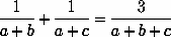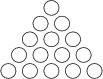## 2005 Qualifying Quiz

You may also be interested in the printable (PDF) format or the LaTeX source code.

1. Here is an unusual way to multiply numbers:

• Head two columns with two positive integers you wish to multiply
• On the next line, write half the previous entry (ignoring remainders) in the first column, and twice the previous entry in the second column. Keep halving and doubling until you get down to 1 in the first column.
• Cross out all rows where the first column has an even number.
• Add all the surviving numbers in the right column. This gives the correct answer!

Example: to compute 54·33, write

```54 33
27 66
13 132
6 264
3 528
1 1056
```
Cross out rows #1 and #4. Add up the surviving numbers on the right:
```66+132+528+1056 = 1782 = 54·33.
```
(a) Does this really always work? Try to give an explanation that would convince the most die-hard skeptic!
(b) The method above is based on multiplying and dividing by 2. Can you invent an analogous method that is based on multiplying and dividing by 3, and explain why it works?

2. Eric and Matthew are visiting the town of Descartes, Québec, and have managed to lose each other. Descartes has five equally spaced streets running east-west, cut by five equally spaced streets running north-south; each of the 16 blocks is a perfect square. Eric is at the southwest corner of town, and decides to look for Matthew by walking towards the northeast corner along a path of shortest length. At each intersection where he has a choice between north and east he chooses which way to go randomly, with both directions equally likely. Meanwhile, Matthew is at the northeast corner, deciding to look for Eric by walking to the southwest corner in exactly the same manner. Both start walking at the same time, and walk at the same speed. What's the probability that they meet?

3. A triangle has sides of length a, b, and c which satisfy the equationDetermine all possible values for the angle opposite the side of length a.

4. Suppose 15 pennies are arranged in a triangle, as in the diagram below.Each coin can be either heads up or tails up. Show that, no matter which pennies are heads up, somewhere in this triangle there must be three pennies which all face the same way (i.e., either all heads up or all tails up) and which form the vertices of an equilateral triangle.

5. Suppose A is a set of one hundred distinct natural numbers with the property that given a, b, c in A (distinct or not), there is a triangle with no angle greater than a right angle, and with sides of lengths a, b, c. What's the smallest possible value for the largest element of A?

6. Three bugs are crawling on the coordinate plane. They move one at a time, and each bug will only crawl in a direction parallel to the line joining the other two.
(a) If the bugs start out at (0,0), (3,0), and (0,3), is it possible that after some time the first bug will end up back where it started, while the other two bugs switch places?
(b) Can the bugs end up at (1,2), (2,5), and (-2,3)?

7. You are a secret agent assigned to carry out classified research on Enemy Government Gadgets (EGGs). Your mission: to determine the highest floor of an office building from which a dropped EGG will survive falling to the ground. Your method: drop EGGs from different floors and see if they break.
(a) The budget is tight at the agency, so you'll only have two EGGs to work with. Once both EGGs break, that's it. Moreover, time is of the essence, and you must guarantee that you can finish your mission within 10 drops. What is the tallest building for which you can be assured of getting a precise answer, even with the worst possible luck?
(b) Generalize the problem: what is the tallest building you can analyze with 2 EGGs if you are allowed k trials? What about k trials and 3 EGGs? k trials and n EGGs?

8. Amazing Amanda is performing a magic trick for her friend Farah. She takes 100 cards, numbered from 1 to 100, and distributes them in three boxes, so that each box contains at least one card. She then leaves the room. Farah chooses two of the boxes, selects a card from each, and tells Amanda their sum. Amazingly, no matter what cards Farah picks, Amanda can immediately tell which two boxes Farah's cards came from! How did Amanda distribute the cards in the boxes? Is there more than one way that works? Describe every possible way she can do it, and prove that there are no others.

9. (a) The integer 9 can be written as a sum of two consecutive positive integers: 9 = 4+5; moreover, it can be written as a sum of (more than one) consecutive positive integers in exactly two ways, namely 9=4+5=2+3+4. Find all integers with this pair of properties.
(b) Find all integers which can be written as a sum of 2004 consecutive positive integers, and which moreover can be written as a sum of (more than one) consecutive positive integers in exactly 2004 ways.

10. In the Game of Euclid, two players start with a pair of distinct positive integers, a and b. The first player subtracts a positive multiple of the smaller integer from the larger one so as to leave a positive remainder. She thus creates a new pair of positive integers. The second player repeats this process with the new pair of integers. The players continue taking turns in this fashion, and the player who creates a pair of equal integers wins. For what pairs (a,b) does the first player have a winning strategy, and what is the strategy?